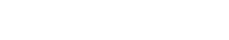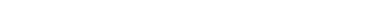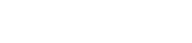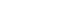# 🌡 Temperature and humidity measurement on Arduino – a selection of methods

To create a home weather station or thermometer, you need to learn how to match the Arduino board and devices for measuring temperature and humidity. Temperature measurement can be managed with a thermistor or a DS18B20 digital sensor, but more sophisticated devices are used to measure humidity — the DHT11 or DHT22 sensors. In this article, we will explain how to measure temperature and humidity with the help of Arduino and these sensors.To create a home weather station or thermometer, you need to learn how to match the Arduino board and devices for measuring temperature and humidity. Temperature measurement can be managed with a thermistor or a DS18B20 digital sensor, but more sophisticated devices are used to measure humidity — the DHT11 or DHT22 sensors. In this article, we will explain how to measure temperature and humidity with the help of Arduino and these sensors.Thermistor measurement

The easiest way to determine the temperature is to use a thermistor. This is a kind of resistor whose resistance depends on the ambient temperature. Thermistors with a positive and negative temperature coefficient of resistance – PTC (also called posistors) and NTC-thermistors, respectively, are distinguished.

On the graph below you see the dependence of resistance on temperature. The dashed line shows the dependence for a thermistor with a negative TCS (NTC) and a thick solid line for a thermistor with a positive TCS (PTC).What do we see here? The first thing that catches your eye is that the PTC thermistor has a broken graph and it will be difficult or impossible to measure a series of temperature values, but for an NTC thermistor, the graph is more or less uniform, though clearly non-linear. What does it mean? Using the NTC thermistor is easier to measure the temperature because it is easier to figure out the function by which its values change.

To convert the temperature to resistance, you can manually remove the values, but this is difficult at home and you need a thermometer to determine the actual values of the ambient temperature. In the datasheets of some components, there is such a table, for example, for a series of NTC-thermistors from Vishay.Then you can arrange the transfer through branching using the function if … else or switch case. However, if there are no such tables in datasheets and it is necessary to calculate the function by which the resistance changes with increasing temperature.

To describe this change there is the Steinhart-Hart equation.where A, B, and C are the thermistor constants determined from measurements of three temperatures with a difference of at least 10 degrees Celsius. At the same time, various sources indicate that for a typical 10 kΩ NTC thermistor they are equal:https://www.bipm.org/utils/common/pdf/ITS-90/Guide-SecTh-Thermistor-Thermometry.pdf

This is a thermistor temperature measurement brochure issued by the Consultative Committee for Thermometry (CCP).

However, the use of such an equation is laborious and in amateur projects is unjustified, so you can use the beta-equation for the thermistor.B is the beta coefficient, it is calculated based on the resistance measurement for two different temperatures. It is indicated either in the datasheet (which is illustrated below), or it is calculated independently.In this case, B is indicated in the form:This means that the coefficient was calculated on the basis of the data obtained in the measurement of resistance at temperatures of 25 and 100 degrees Celsius, this is the most common variant. Then it is calculated by the formula:

B = (ln (R1) – ln (R2)) / (1 / T1 – 1 / T2)

A typical circuit for connecting a thermistor to a microcontroller is shown below.Here R1 is a fixed resistor, the thermistor is connected to the power supply, and the data is removed from the midpoint between them, the diagram conventionally indicates that the signal is fed to the output A0 – this is the Arduino analog input.To calculate the resistance of the thermistor, you can use the following formula:

R thermistor = R1⋅ ((Vcc / Voutput) −1)

To translate into a language understandable for Arduino, you need to remember that the Arduino has a 10-bit ADC, which means the maximum digital value of the input signal (voltage 5V) will be 1023. Then by convention:

• Dmax = 1023;
• D is the actual value of the signal.

Then:

Thermistor = R1⋅ ((Dmax / D) −1)

Now we use this to calculate the resistance and then calculate the thermistor temperature using the beta equation in the Arduino programming language. The sketch will be:DS18B20

Even more popular for measuring temperature with. Arduino found a DS18B20 digital sensor. It communicates with the microcontroller via the 1-wire interface, you can connect several sensors (up to 127) to one wire, and in order to access them, you will have to find out the ID of each of the sensors.

Note: You must know the ID even if you use only 1 sensor.The connection diagram of the ds18b20 sensor to Arduino looks like this:There is also a parasitic power mode – its wiring diagram looks like this (you need two wires instead of three):In this mode, correct operation is not guaranteed when measuring temperatures above 100 degrees Celsius.

The DS18B20 digital temperature sensor consists of a whole set of nodes, like any other CIMS.

To work with it, you need to download the Onewire library for Arduino, and for the sensor itself, it is recommended to use the DallasTemperature library.This sample code demonstrates the basics of working with 1 temperature sensor, the result in degrees Celsius is output via the serial port after each read.

DHT11 and DHT22 – humidity and temperature sensorsThese sensors are popular and are often used to measure humidity and ambient temperature. In the table below we indicate their main differences.

 DHT11 DHT22 Determination of humidity in the range 20-80% 0-100% Accuracy of measurements 5% 2-5% Temperature determination from 0 ° C to + 50 ° C from -40 ° C to + 125 ° C Accuracy of measurements 2.5% +- 0.5 Degrees Celsius Poll Frequency 1 per second 1 per 2 secondsThe connection diagram is quite simple:

• 1 conclusion – power;
• 2 conclusion – the data;
• 3 conclusion – not used;
• 4 pin – common wire.

If you have a sensor in the form of a module, it will have three leads, but no resistor is required, it is already soldered on the board.To work, we need the DHT.h library. It is not in the standard set, so it needs to be downloaded and installed in the libraries folder in the folder with the Arduino IDE. It supports all sensors of this family:

• DHT 11;
• DHT 21 (AM2301);
• DHT 22 (AM2302, AM2321).

Example of using the library:Conclusion

Nowadays, to create your own station for measuring temperature and humidity is very easy thanks to the Arduino platform. The cost of such projects is 3-4 hundreds of rubles. For autonomous work, rather than data output to a computer, a symbolic display can be used (we described them in a recent article), then you can build a portable device for use both at home and in a car. Write in the comments what else you would like to know about simple homemade articles on Arduino!